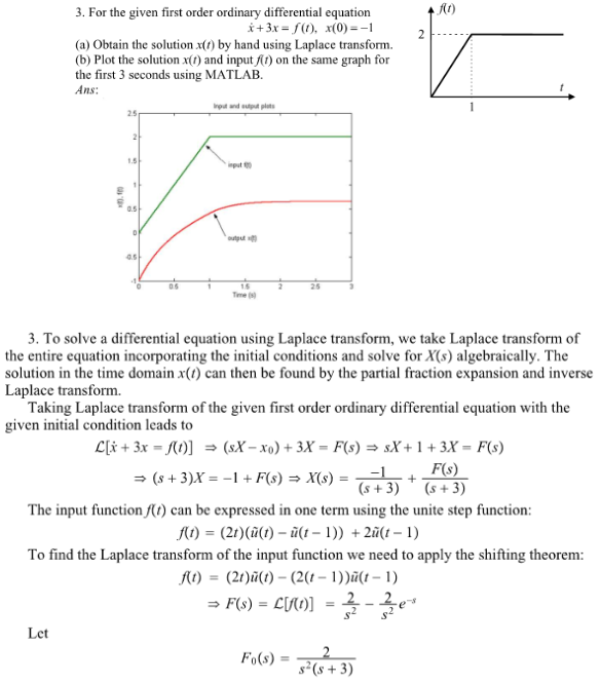For the given first order ordinary differential equation xdot+3x=f(t), x(0)=-1, (a) Obtain the solution x(t) by hand using Laplace transform. (b) Plot the solution x(t) and input f(t) on the same graph for the first 3 seconds using MATLAB.For the given first order ordinary differential equation xdot+3x=f(t), x(0)=-1, (a) Obtain the solution x(t) by hand using Laplace transform. (b) Plot the solution x(t) and input f(t) on the same graph for the first 3 seconds using MATLAB.

System Dynamics Page 2 dynamics dynamics dynamics dynamics dynamics dynamics dynamics System dynamics Page 3